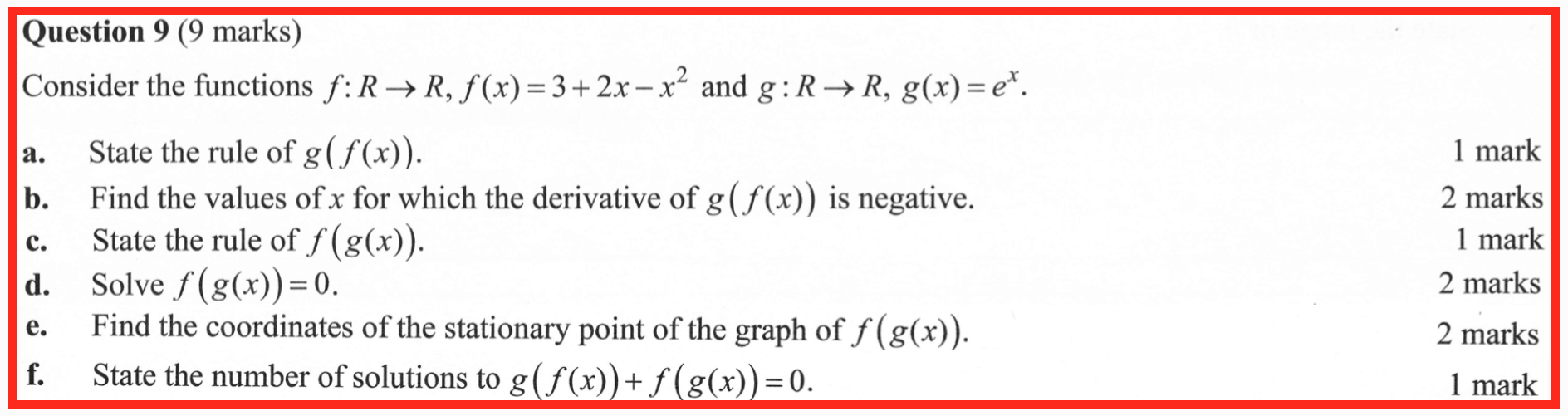## WitCH 31: Decomposing

We have a short Specialist post coming, and we’ll have more to write on the 2019 VCE exams once they’re online. But, for now, one more Mathematical Methods WitCH, from the 2019 (calculator-free) Exam 1:## Update (04/07/20)

The main crap here, of course, is part (f): as commenter John Friend puts it, what the hell is this question supposed to be testing? And, sure, the last part of the last question on an exam is allowed to be a little special, but one measly mark? Compared to the triviality of the rest of the question?

Of course, students bombed part (f). The examination report indicates that 19% of student correctly answered that there is one solution to the equation; as suggested by commenter Red Five, it’s also a pretty safe bet that the majority of students who got there did so with a Hail Mary guess. (It should be added, the students didn’t do swimmingly well on the rest of Question 9, the CAS-lobotomising having working its usual magic.)

OK, so what did examiners expect for that one measly mark? We’ll get to a reasonable solution below, but let’s first consider some unreasonable solutions.

Here is the examination report’s entire commentary on Part (f):

g(f(x) + f(g(x)) = 0 has exactly one solution.

This question was not well done. Few students attempted to draw a rough sketch of each equation and use addition of ordinates.

Gee, thanks. Drawing a “rough sketch” of either of these compositions is anything but trivial. For one measly mark. We’ll look at sketching aspects of these graphs below, but let’s get on with another unreasonable solution.

Given the weirdness of part (f), a student might hope that parts (a)-(e) provide some guidance. Let’s see.

Part (b) (for which the examination report contains an error), gets us to conclude that the compositionhas negative derivative when x > 1.

Part (c) leads us to the compositionhaving x-intercept when x = log(3).

Finally, Part (e) gives us that the composition f(g(x)) has the sole stationary point (0,4). How does this information help us with Part (f)? Bugger all.

So, what if we include the natural implications of our previous work? That gives us something like the following:Well, um, great. We’re left still hunting for that one measly mark.

OK, the other parts of the question are of little help, and the examiners are of no help, so what do else do we need? There are two further pieces of information we require (plus the Intermediate Value Theorem). First, note thatSecondly, note thatif x is huge.

Then, given we know the slopes of the compositions, we can finally complete our rough sketches:Now, let’s write S(x) for our sum function g(f(x)) + f(g(x)). We know S(x) > 0 unless one of our compositions is negative. So, the only place we could get S = 0 is if x > log(3). But S(log(3)) > 0, and eventually S is hugely negative. That means S must cross the x-axis (by IVT). But, since S is decreasing for x > 1, S can only cross the axis once, and S = 0 must have exactly one solution.

We’ve finally earned our one measly mark. Yay?